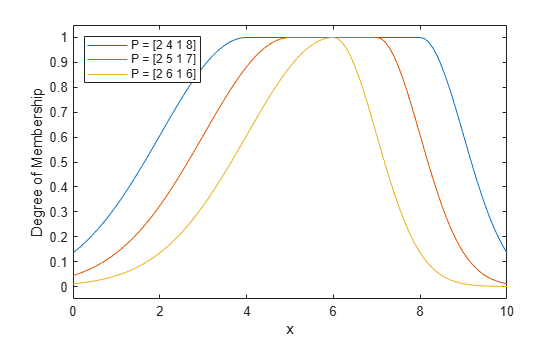# gauss2mf

Gaussian combination membership function

## Syntax

``y = gauss2mf(x,params)``

## Description

This function computes fuzzy membership values using a combination of two Gaussian membership functions. You can also compute this membership function using a `fismf` object. For more information, see fismf Object.

example

````y = gauss2mf(x,params)` returns fuzzy membership values computed using a combination of two Gaussian membership functions computed. Each Gaussian function defines the shape of one side of the membership function and is given by:$f\left(x;\sigma ,c\right)={e}^{\frac{-{\left(x-c\right)}^{2}}{2{\sigma }^{2}}}$To specify the standard deviation, σ, and mean, c, for each Gaussian function, use `params`.Membership values are computed for each input value in `x`.```

## Examples

collapse all

Specify input values across the universe of discourse.

`x = 0:0.1:10;`

Evaluate several membership functions for the input values.

```y1 = gauss2mf(x,[2 4 1 8]); y2 = gauss2mf(x,[2 5 1 7]); y3 = gauss2mf(x,[2 6 1 6]);```

Plot the membership function.

```plot(x,y1,x,y2,x,y3) xlabel('x') ylabel('Degree of Membership') ylim([-0.05 1.05]) legend('P = [2 4 1 8]','P = [2 5 1 7]','P = [2 6 1 6]',... 'Location','northwest')```## Input Arguments

collapse all

Input values for which to compute membership values, specified as a scalar or vector.

Membership function parameters, specified as the vector [σ1 c1 σ2 c2]. Here:

• σ1 and c1 are the standard deviation and mean of the left Gaussian function, respectively.

• σ2 and c2 are the standard deviation and mean of the right Gaussian function, respectively.

When c1c2, the `gauss2mf` function reaches a maximum value of `1` over the range [c1, c2].

Otherwise, when c1> c2, the maximum value is less than one.

## Output Arguments

collapse all

Membership value, returned as a scalar or a vector. The dimensions of `y` match the dimensions of `x`. Each element of `y` is the membership value computed for the corresponding element of `x`.

## Alternative Functionality

### `fismf` Object

You can create and evaluate a `fismf` object that implements the `gauss2mf` membership function.

```mf = fismf("gauss2mf",P); Y = evalmf(mf,X);```

Here, `X`, `P`, and `Y` correspond to the `x`, `params`, and `y` arguments of `gauss2mf`, respectively.

## Version History

Introduced before R2006a

expand all